211213 Epaper

 A Section B SectionC Section D Section211212 Epaper

 A Section B SectionC Section D Section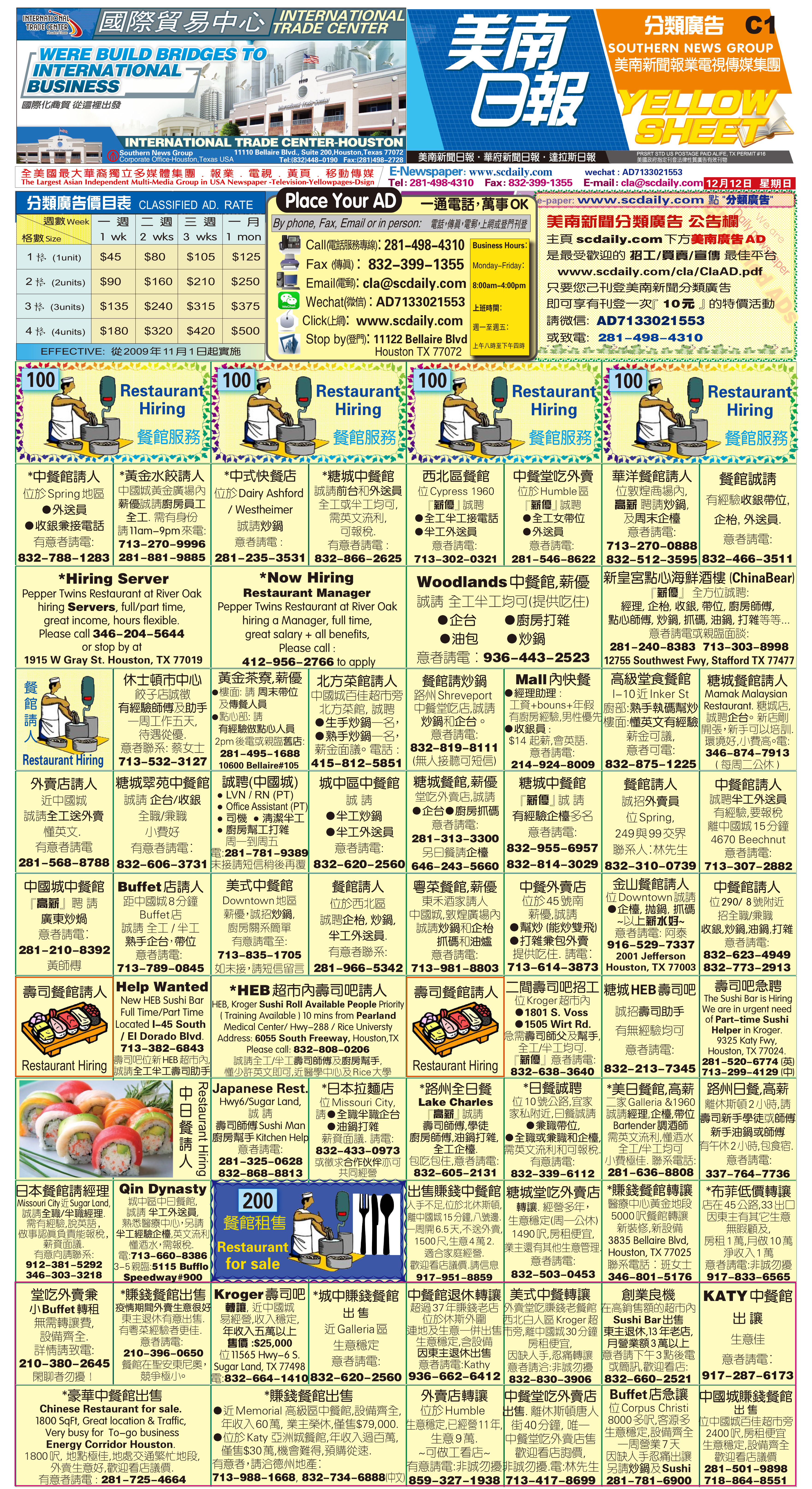211210 Epaper

 A Section B SectionC Section D Section211209 Epaper

 A Section B SectionC Section D Section211208 Epaper

 A Section B SectionC Section D Section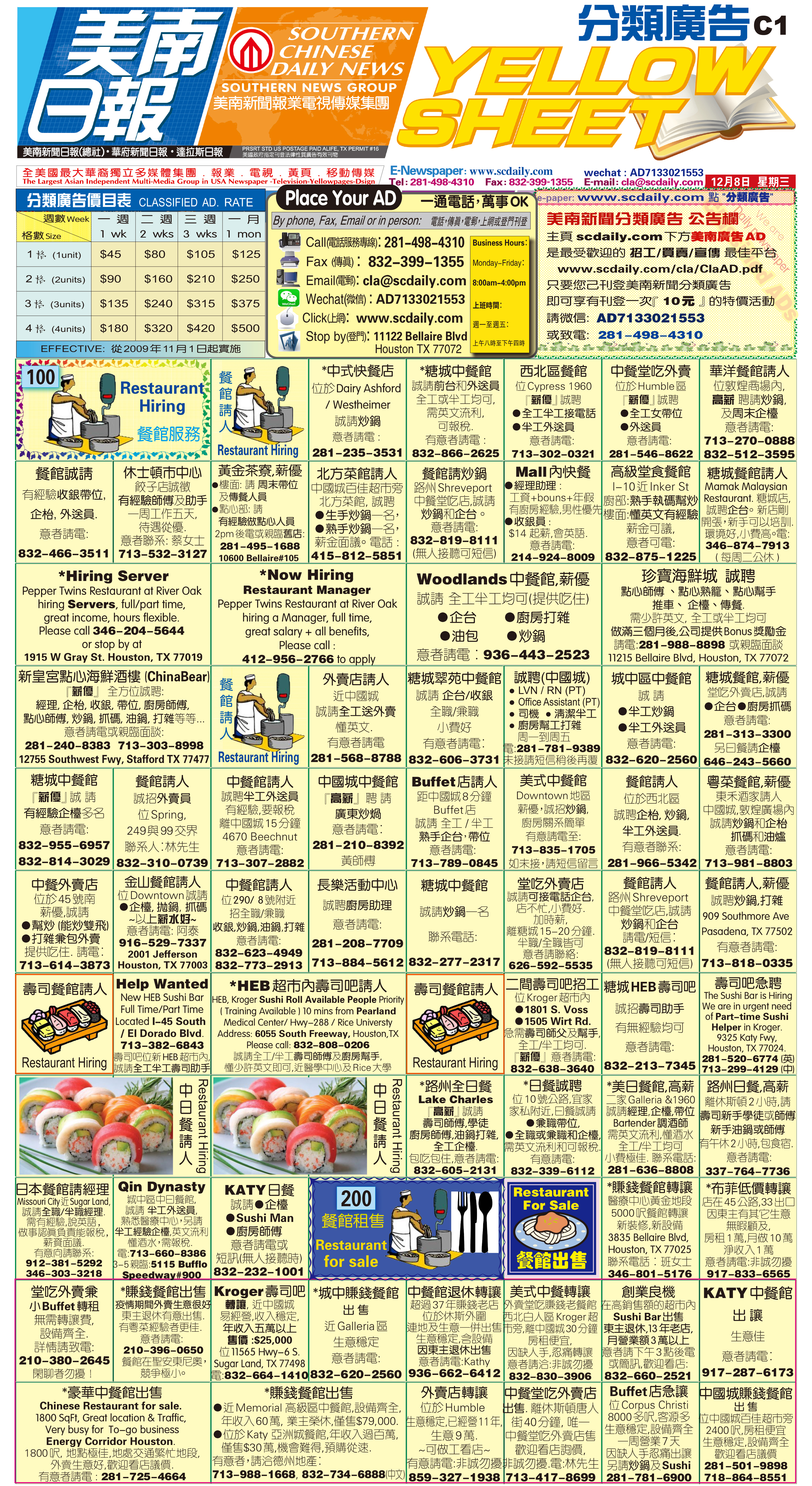211207 Epaper

 A Section B SectionC Section D Section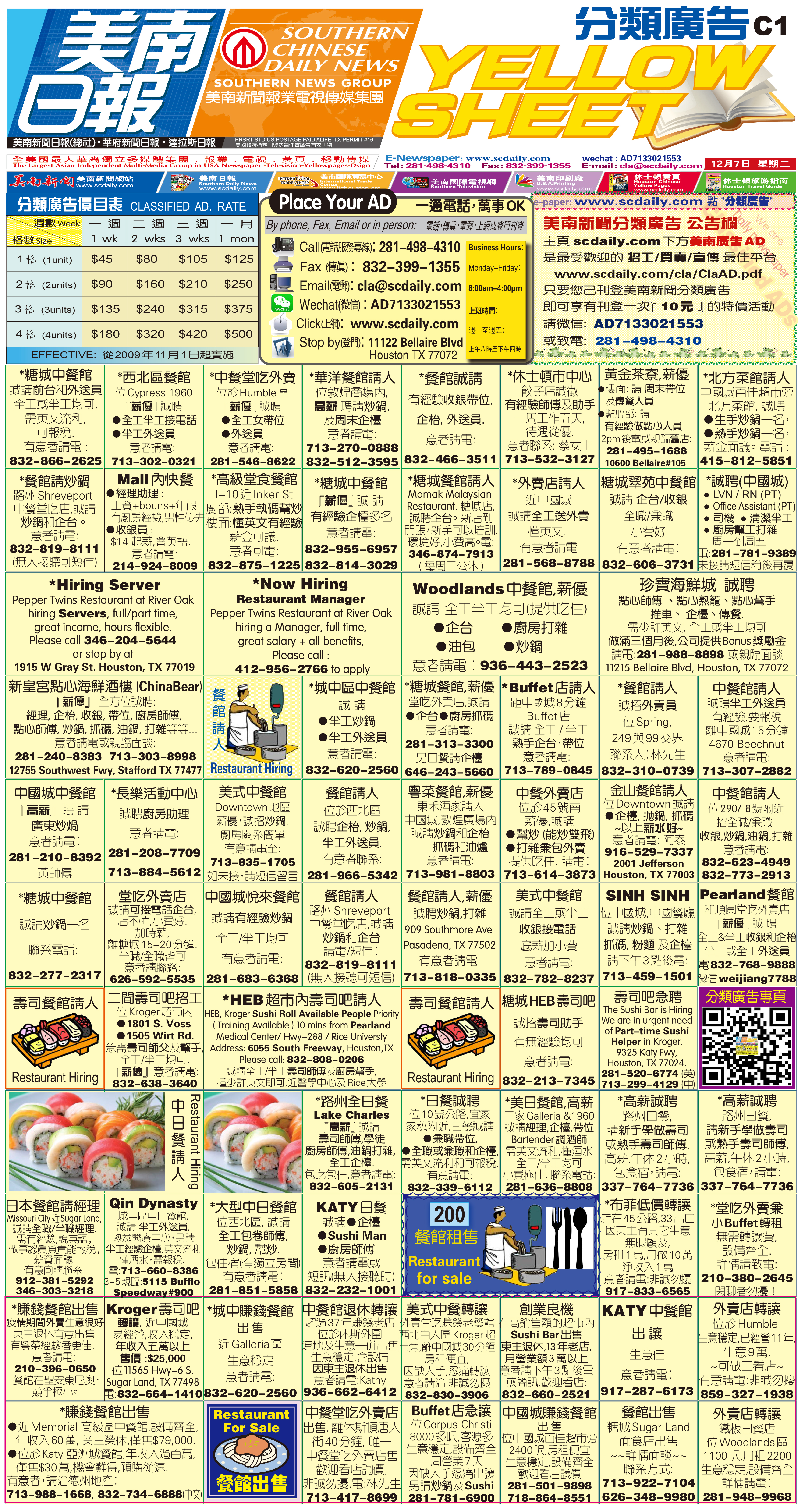211206 Epaper

 A Section B SectionC Section D Section211205 Epaper

 A Section B SectionC Section D Section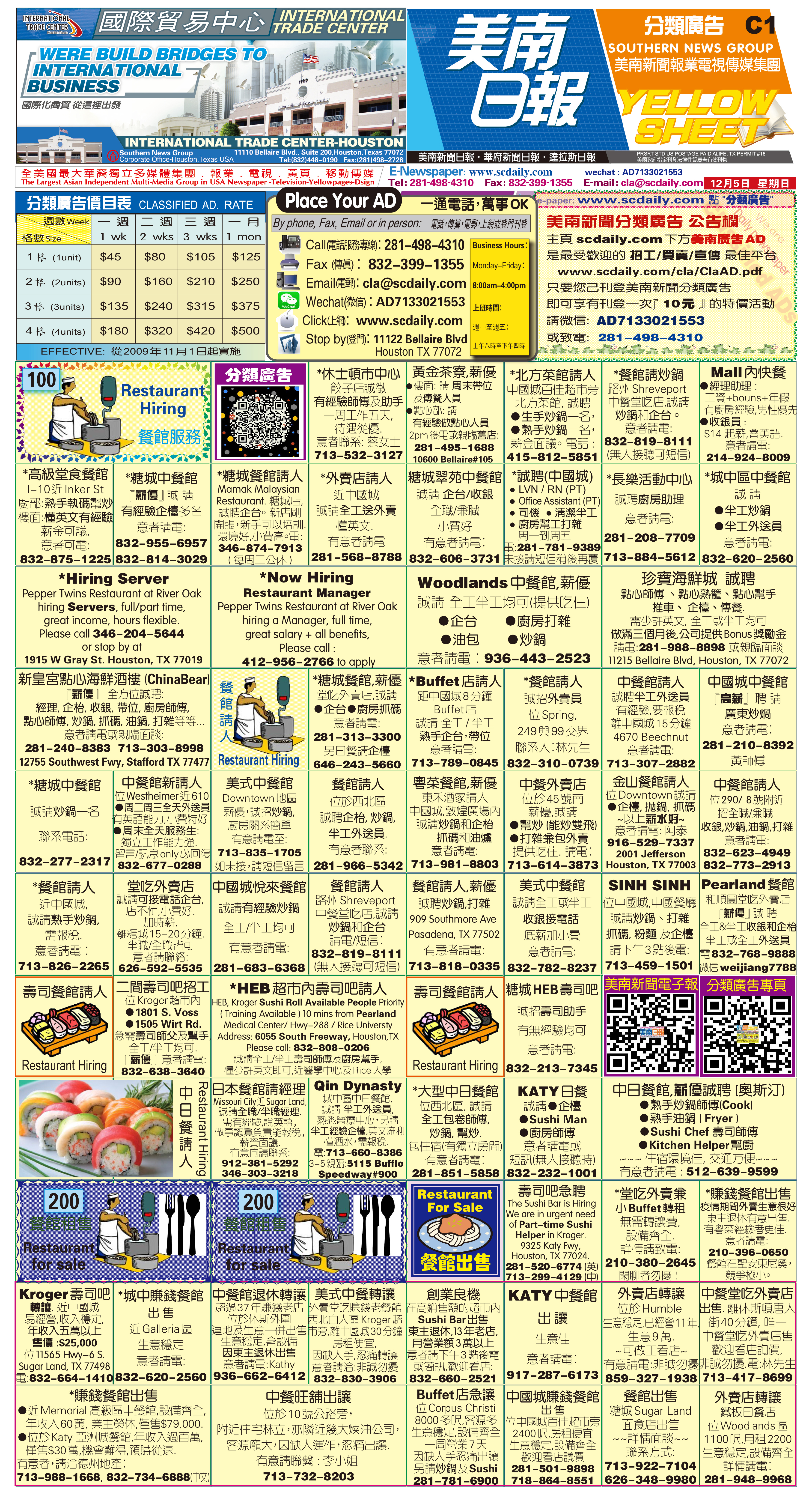211203 Epaper

 A Section B SectionC Section D Section211204 Epaper

 A Section B SectionC Section D Section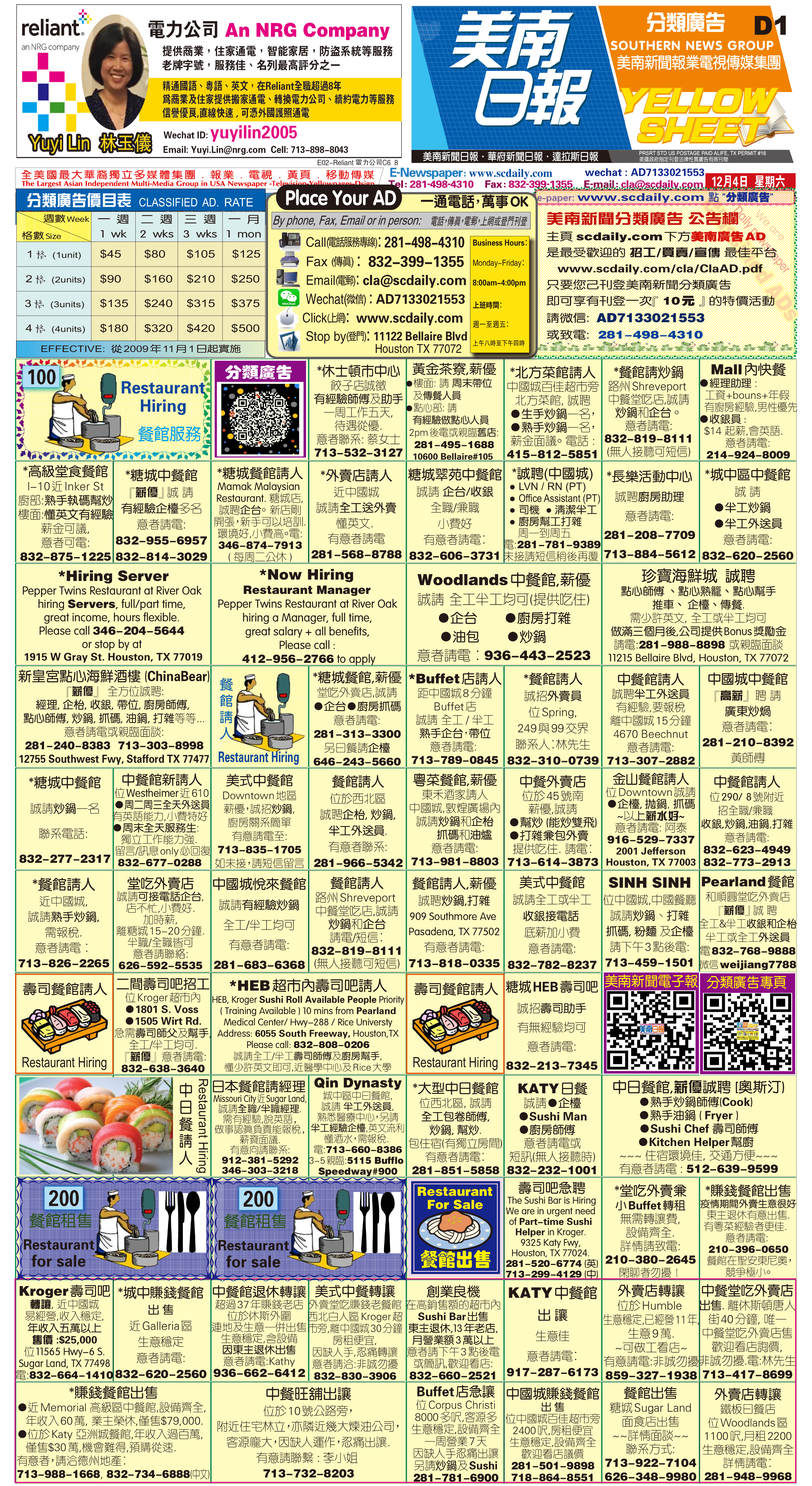211202 Epaper

 A Section B SectionC Section D Section